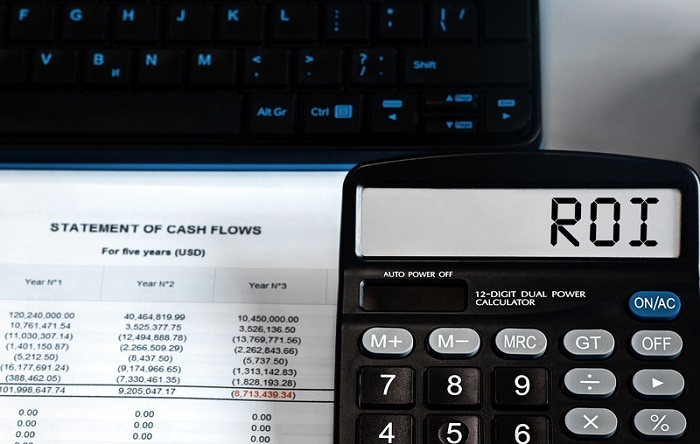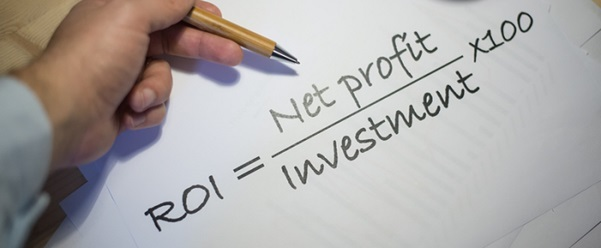# What Is Return On Investment

## Introduction

Return on Investment (ROI) lets you determine the profit or loss generated when you invest money in a company or asset/venture. This metric expresses the efficiency and performance of the investor’s investment in a company over the period of time.One can use this metric, frequently stated as a percentage or ratio, to convey the gain or financial returns or losses per se.

## What is Return on Investment?

Return on investment is a performance metric that assesses an investment's effectiveness or profitability. One can also use it to compare the efficacy of investments. ROI quantifies the amount of return on a particular investment in relation to its cost.

The amount you obtain from an investment is the net profit or cost of the investment minus its current value. An investment’s loss or net profit is divided by the investment's cost to calculate the return on investment.

## Calculation of Return on Investment

To determine the return on investment, divide the net profit from an investment by the cost of the investment, then multiply the result by 100. The outcome is expressed as a percentage. The formula goes as follows −

$$\mathrm{ROI =\frac{Net\:Profit\:or \:Loss}{Cost\:of\:Investment}\times 100}$$

$$\mathrm{ROI =\frac{Present\:Value – Cost\:of\:Investment }{Cost\:of\:Investment}\times 100}$$

ROI is computed as a percentage by taking total returns and total costs into account. In numerator, you include net returns, and in the denominator, you take cost of investment.

When it comes to present value computation, investments that could yield interest in the future are considered here.

The percentage form of ROI as an outcome makes it easier for the companies to compare their competitive standing in their respective line of business. One can’t figure out the growth and performance of the company with apples to oranges comparison, and ROI eliminates that hindrance and aids in attaining accurate results.

For example, Person A buys shares for INR 1,000 and, one year later, sells them for INR 1,500. In this instance, the investment's net profit (current value - cost) is INR 500 (INR 1,500 - INR 1,000), and the return on investment is:

$$\mathrm{\frac{INR500 (Net\:Profit)}{INR1000 (Cost\:of\:Investment)}\times 100=50\% \:ROI}$$

## Understanding the concept of Return on Investment

ROI is the basic criterion of an investment's profitability. It might be the return on investment (ROI) from a stock purchase, the ROI a business anticipates from expanding manufacturing, or the ROI produced in a real estate deal.

Simply put, the return on investment of an investment is net positive if it is likely to be profitable. These indications, however, can assist investors in deciding which possibilities to pursue or dismiss if other chances with greater ROIs are available. Similar to negative ROIs, which indicate a net loss, investors should steer clear of them.

For example, an ecommerce store invested INR 5,000 on an online marketing campaign. After a few days, he discovered that the campaign increased revenue by INR 10,000.

So, the return on investment would be −

$$\mathrm{\frac{Rs\:10000 (Net\:Profit)- Rs\: 5000 (Cost\:of\:Investment)}{Rs\:5000 (Cost\:of \:Investment)}\times 100=100\% ROI}$$

ROI is used to evaluate a diverse range of indicators that contribute to an organization's profitability. The organization should measure entire returns and total expenditures to determine ROI as accurately as possible.

Positive return percentages in ROI calculations indicate that the company, or the ROI metric being assessed, is profitable. On the other hand, a negative ROI percentage shows that the company or the metric it is being evaluated against owes more money than it is bringing in. In other words, if the proportion is positive, returns outweigh costs. If the percentage is lower than zero, the investment is losing money.

## Why is ROI used?

Comparing ROI to the initial cost helps assess different investment decisions. When evaluating current or past investments, businesses frequently employ ROI estimates.People may use the ROI to evaluate their investments and contrast one investment in their portfolios with another. The method holds for both a stock holding and a financial interest in a small business.

### Good ROI

What constitutes a "good" ROI will vary depending on the investor's risk tolerance level and the time it takes for an investment to pay off.

Given all other factors being equal, risk-averse investors will probably accept lower returns in exchange for incurring fewer risks, and vice-versa. Additionally, for an investment to appeal to investors, it often needs a better return on investment (ROI).

### Benefits of ROI• Simple Calculation: Tracking effectiveness and profitability is made simple by the ROI formula, which only needs a few inputs to produce the end result.

• Results: A positive number denotes a gain, whereas a negative number denotes a loss.

• Flexibility: ROI applies to a range of investments, including stock purchases, automobile purchases, house improvements, and more.

### Limitations of ROI

• An investment choice is not necessarily preferable if the ROI is larger.

• ROI assessments vary from company to company.

• Errors can happen at any time.

• Unable to factor time into the calculation.

## Conclusion

A common profitability statistic used to assess an investment's performance is the return on investment (ROI). Dividing an investment's net profit/loss by the cost of investment results an ROI. One may use this metric to rate investments in various projects or assets and create comparisons. ROI does not account for the opportunity costs of investing.

## FAQs

Q1. Why is ROI important?

Ans. Return on investment is significant when considering what technology can do for a business. ROI determines if an investment, internal or external, was successful. Once you evaluate an investment's success, you may adjust to raise it in the future.

Q2. How can you use ROI?

Ans. Regular investors may use ROI to review their portfolios, and they can also use it to evaluate nearly any kind of expense. Remember that ROI cannot eliminate risk or uncertainty; it can only be as accurate as the data you input into the calculation.

You still need to account for the possibility of your predictions of net earnings being too optimistic or pessimistic when using ROI to make investment decisions in the future.

Q3. What is an ROI calculator?

Ans. An ROI calculator estimates the profit or loss of your investment. Before taking any investment decision in a project, use this metric as a tool to learn the bigger picture of that particular deal.

Q4: How can businesses benefit from ROI?

Ans. ROI calculations are not meant to be accurate measurement techniques, but rather approximation methods. More precise estimates are usually beneficial, although some inaccuracy is expected with ROI. Assessing the return on any deal aids in identifying effective company strategies.

Updated on: 05-Apr-2023

138 Views